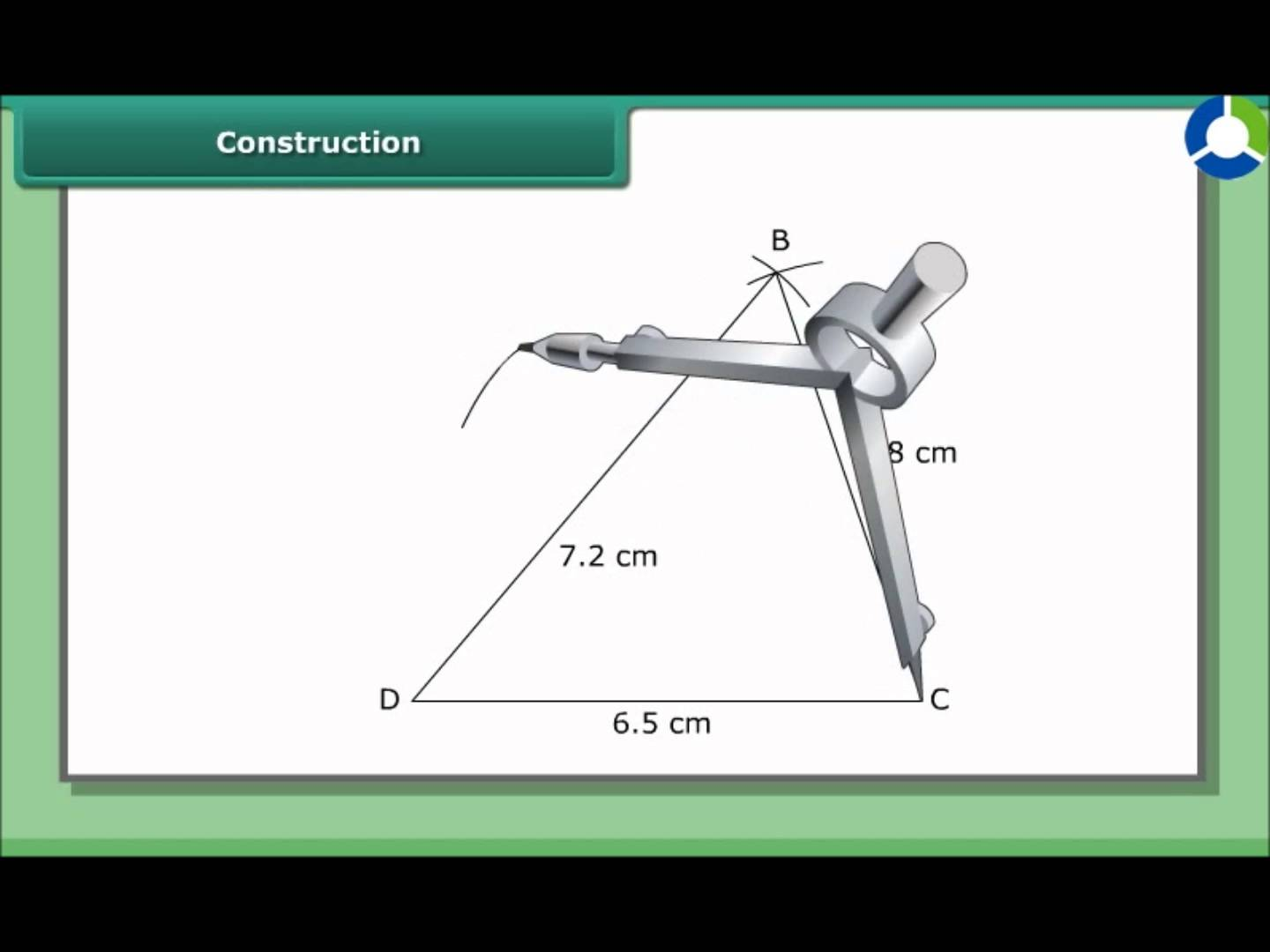# Practical Geometry worksheet for class 7## myCBSEguide App

CBSE, NCERT, JEE Main, NEET-UG, NDA, Exam Papers, Question Bank, NCERT Solutions, Exemplars, Revision Notes, Free Videos, MCQ Tests & more.

## Practical Geometryworksheet for class 7 Important Topics

• Construction of a line parallel to a given line, through a point not on the line
• Construction of Triangles : An idea
• Constructing a triangle when the lengh of its sides are known (SSS Criterion)
• Constructing a triangle when the length its two sides and the measure of the angle between them are known (SAS Criterion)
• Constructing a triangle when the measure of two of its angles and the length of the side included between them is given (ASA Criterion)
• Constructing a Right-Angled Triangle when the length of one leg and its Hypotenous are given (RHS Criterion)## Some important Facts about Practical Geometryworksheet for class 7

In this Chapter, we looked into the methods of some ruler and compasses constructions.

1. Given a line l and a point not on it, we used the idea of ‘equal alternate angles’ in a transversal diagram to draw a line parallel to l. We could also have used the idea of ‘equal corresponding angles’ to do the construction.
2. We studied the method of drawing a triangle, using indirectly the concept of congruence of triangles.

The following cases were discussed:

1. SSS: Given the three side lengths of a triangle.
2. SAS: Given the lengths of any two sides and the measure of the angle between these sides.
3. ASA: Given the measures of two angles and the length of side included between them.
4. RHS: Given the length of hypotenuse of a right-angled triangle and the length of one of its legs.

## NCERT class 7Mathematics Solved Worksheets

• Chapter 1 – Integers
• Chapter 2 – Fractions and Decimals
• Chapter 3 – Data Handling
• Chapter 4 – Simple Equations
• Chapter 5 – Lines and Angles
• Chapter 6 – Practical Geometry
• Chapter 7 – The Triangle and its Properties
• Chapter 8 – Congruence of triangles
• Chapter 9 – Comparing Quantities
• Chapter 10 – Rational numbers
• Chapter 11 – Perimeter and Area
• Chapter 12 – Algebraic Expressions
• Chapter 13 – Exponents and Powers
• Chapter 14 – Symmetry
• Chapter 15 – Visualizing Solid Shapes

## CONSTRUCTION OF TRIANGLES

It is better for you to go through this section after recalling ideas on triangles, in particular, the chapters on properties of triangles and congruence of triangles.

You know how triangles are classified based on sides or angles and the following important properties concerning triangles:

1. The exterior angle of a triangle is equal in measure to the sum of interior opposite angles.
2. The total measure of the three angles of a triangle is 180°.
3. Sum of the lengths of any two sides of a triangle is greater than the length of the third side.
4. In any right-angled triangle, the square of the length of hypotenuse is equal to the sum of the squares of the lengths of the other two sides

To download Printable worksheets for class 7 Mathematics and Science; do check myCBSEguide app or website. myCBSEguide provides sample papers with solution, test papers for chapter-wise practice, NCERT solutions, NCERT Exemplar solutions, quick revision notes for ready reference, CBSE guess papers and CBSE important question papers. Sample Paper all are made available through the best app for CBSE students and myCBSEguide website.